# ±500 kV宝鸡换流站无功补偿的优化研究Optimization Research on Reactive Compensation of ±500 kV Baoji Converter Station

• 全文下载: PDF(5049KB)    PP.73-83   DOI: 10.12677/MOS.2019.82010
• 下载量: 250  浏览量: 689   科研立项经费支持

According to the actual situation of Baoji Converter Station, starting from the reactive power de-mand system and combining the current ability of the system to provide reactive power, the sim-ulation analysis of the compensation mode in the station is carried out to find the defects of the existing switching mode and reactive compensation device through theoretical calculation and analysis, which proposes two schemes to improve the existing reactive compensation switching strategy and add SVC (TSC + TCR) reactive compensation device. The two schemes are modeled and simulated respectively, and the simulation results verify the feasibility of the scheme. Comparing before and after the introduction of SVC (TSC + TCR), the most suitable scheme for reactive compensation of Baoji Converter Station is obtained, which provides a basis for the improvement of reactive compensation device of Baoji Converter Station.

1. 引言

2. 宝鸡换流站无功补偿的配置

3. 宝鸡换流站无功补偿的现状

3.1. 滤波器组和电容补偿的投切原则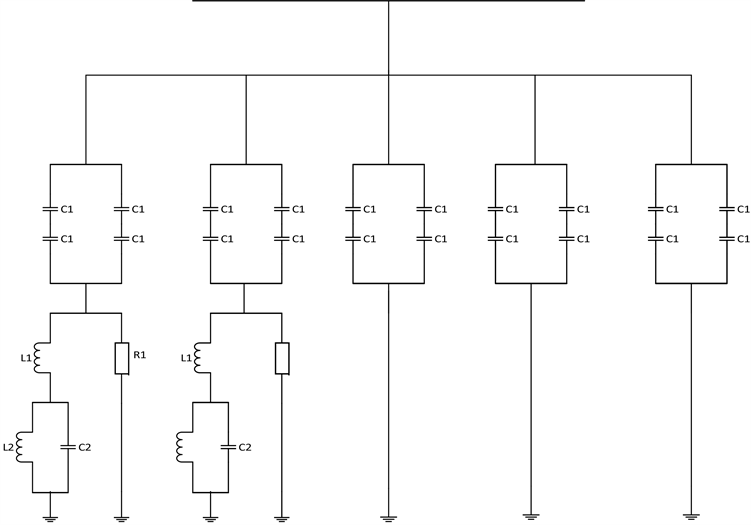Figure 1. Circuit structure diagrams of capacitor and AC filters

3.2. 暂态电压变化率要求

$\Delta U=\frac{\Delta {Q}_{filter}}{{s}_{d}-\sum {Q}_{filter}}$ (1)

3.3. 现有无功装置补偿

3.3.1. 无功补偿仿真分析

1) 330 kV交流场的拓扑图(图2)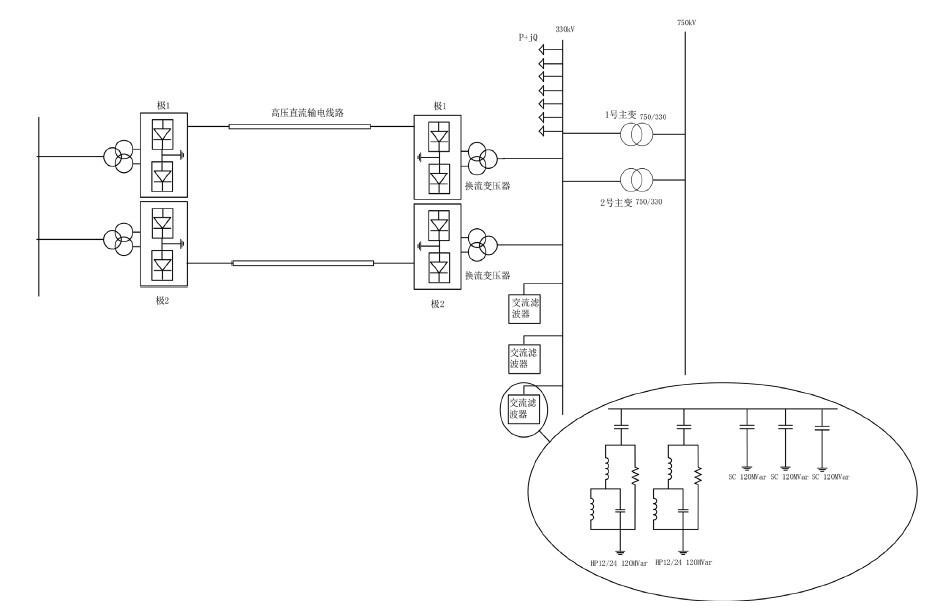Figure 2. 330 kV topology

2) 仿真结果分析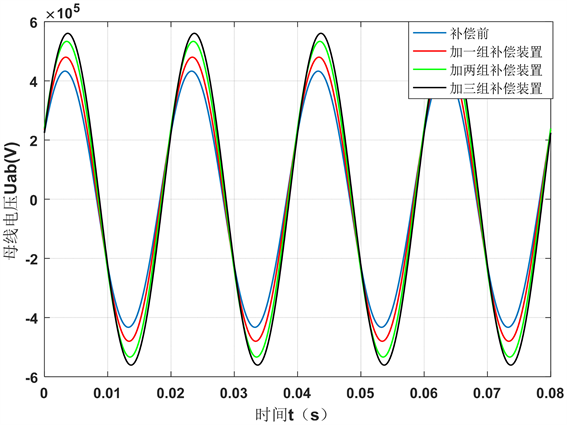Figure 3. Waveform diagram of 330 kV bus-voltage Uab after reactive power with different capacities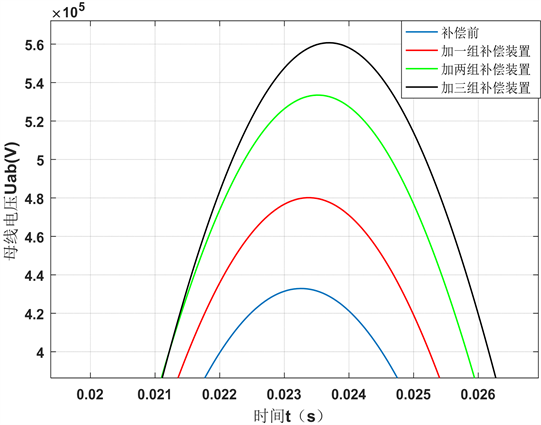Figure 4. Waveform after amplification of Figure 3’s marked part

3.3.2. 现有无功补偿装置存在的问题

4. 宝鸡换流站无功补偿的优化

4.1. 投切策略的改进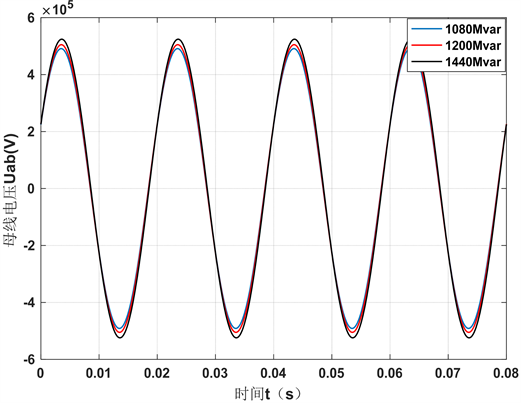Figure 5. Waveform diagram of 330 kV bus-voltage Uab after reactive power with different capacities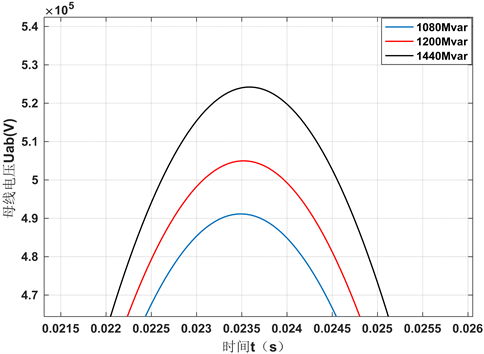Figure 6. Waveform after amplification of Figure 5’s marked part

4.2. 增设SVC无功补偿装置

4.2.1. SVC (TSC + TCR)仿真图和控制模块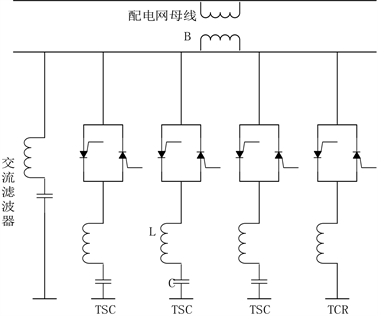Figure 7. SVC (TSC + TCR) three-phase circuit schematic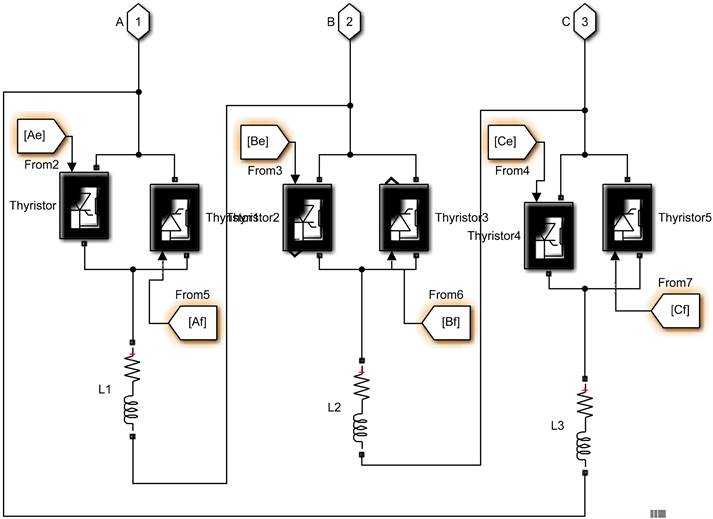(a) TCR的仿真模型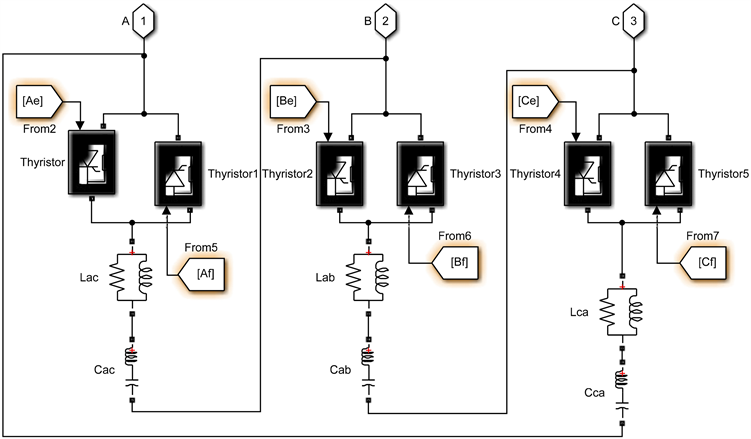(b) TSC的仿真模型

Figure 8. Simulation model of TSC + TCR type SVC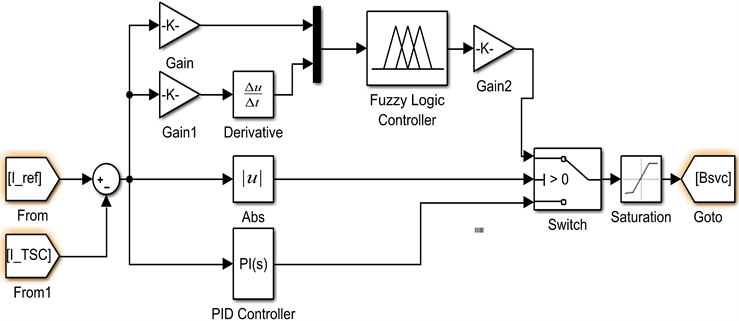Figure 9. Control module

4.2.2. 仿真分析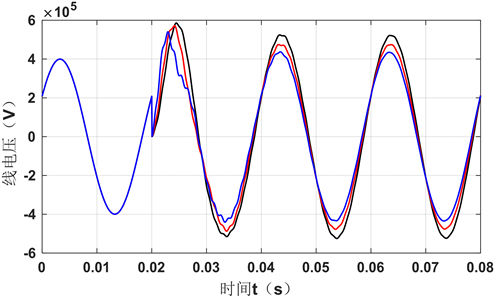Figure10. Line voltage waveform diagram under different capacities

4.3. 优化前后性能比较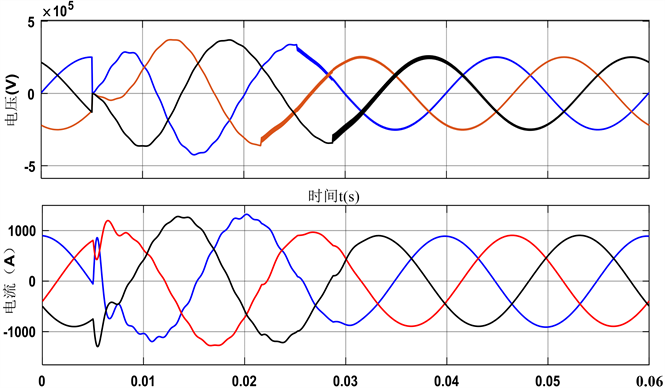Figure 11. 330 kV bus-voltage and current waveform diagram when AC filter compensation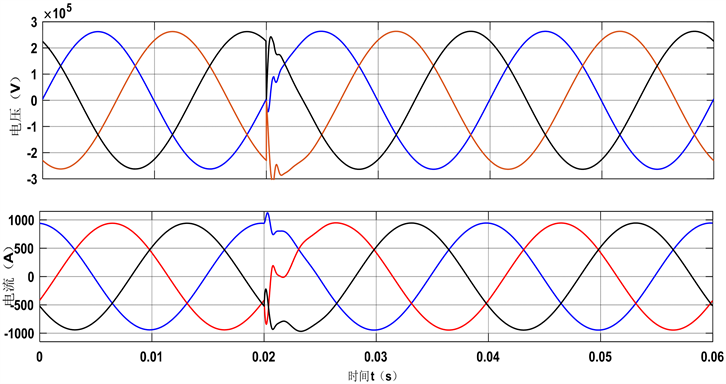Figure 12. 330 kV bus-voltage and current waveforms when SVC compensation is added

5. 结束语

  张忠华, 胡杰. SVC与固定无功补偿设备协调控制实用效果分析[J]. 吉林电力, 2015, 43(6): 28-31.  吴蓉. 变电站无功补偿策略研究[D]: [硕士学位论文]. 杭州: 浙江大学, 2011.  时鑫. ±660 kV直流输电系统换流站无功补偿方案优化设计与仿真[D]: [硕士学位论文]. 哈尔滨: 哈尔滨理工大学, 2013.  文继锋, 陈松林, 李海英, 张宏波, 刘伟. 交流滤波器保护配置和实现[J]. 电力系统自动化, 2006, 30(2): 109-112.  岳彬. SVC在电力系统中的应用及其对电力系统稳定性的影响[D]: [硕士学位论文]. 南京: 南京理工大学, 2012.  文卫兵, 刘菲, 官澜, 康鹏, 胡君慧, 赵娟. 特高压交流变电站无功补偿装置直接接入500 kV母线方案研究[J/OL]. 中国电力: 1-7, 2019-05-27.  李辉, 徐浩, 郭思源, 吴晋波, 刘海峰, 欧阳帆. ±800 kV湘潭换流站交流滤波器投切策略及优化[J]. 电力电容器与无功补偿, 2017, 38(3): 36-42.  陈宇飞. 静止无功补偿装置动态无功补偿技术探讨[J]. 供用电, 2007, 24(3): 60-62.  陈建军. 变电站10 kV动态无功补偿方案设计[J]. 华北电力技术, 2007(4): 52-54.  刘福宁. SVG与SVC无功补偿容量的仿真对比研究[J]. 机电信息, 2017(24): 25+27.  李诚. 风力发电系统静止无功发生器在线监测系统的设计与实现[D]: [硕士学位论文]. 上海: 华东理工大学, 2016.  于琳琳, 刘君, 裴彦东, 赵睿. 静止无功补偿装置(SVC)在东鞍山220 kV变电站的应用[J]. 电气应用, 2013, 32(S1): 479-481+495.  Lu, L., Wang, N.B., Ma, Y.H. and He, S. (2013) Comprehensive Solution for Jiuquan Large-Scale Wind Power Integration. Electric Power Construction, 34, 7-11.  Wang, H. (2011) Research on Reactive Power and Voltage Regulation of DFIG Based Farms. M.A Dissertation, Department of Electric Engineering, North China Electric Power University, Beijing.